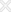Upcoming Batches
Enquiry Form### Basic Course Objective

In this course, you will develop a 3-statement model completely from scratch, inputting historical data and assumptions to project out financial statements using step-by-step instruction on selecting, locating, and developing appropriate projection drivers. At completion, you will have developed a complete and comprehensive three-statement model using various supporting schedules. By the end of the course, trainees will gain skills that lay the foundation for many other modeling methodologies.### What Candidates Learn

• Using keys instead of mouse in excel
• Sorting Data and using advanced filters to overcome the limitations of filters
• Cell Freeze, Row Freeze, Column Freeze
• Using conditional formatting in excel and making the data look more meaty
• Linkages used for Financial Modeling and Valuations Program
• Simple excel formulas as sum, product, division, multiplications, paste special, concatenate
• Vlook Up/H look up usage of data
• Match Function
• Combination of multiple functions in a problem as Vlook +Match, Index+Match, VlookUp and If
• CAGR Calculation
• Transpose function
• Usage of IF function
• Table functions
• Pivot Tables
• IRR Calculation
• Cell Referencing
• Interest functions as EMI calculator,
• Sum if, Count if, Sumifs
• SumProduct Functions
• Multiple usage of Vlook Function

### Got a Question?

We're here to help. Check out our FAQs, send us an email or call us at +91-9953729651